# Samacheer Kalvi 6th Maths Solutions Term 1 Chapter 1 Numbers Ex 1.2

## Tamilnadu Samacheer Kalvi 6th Maths Solutions Term 1 Chapter 1 Numbers Ex 1.2Question 1.
Fill in the blanks with > or < or =

1. 48792 __ 48972
2. 1248654 __ 1246854
3. 658794 __ 658794

Solution:

1. 48792 < 48972
[Hint: Open side can hold large number 7 < 9
2. 1248654 > 1246854
Hint: 8 > 6
3. 658794 = 658794

Question 2.
Say True or False

1. The difference between the smallest number of seven digits and the largest number of six digits is 10.
2. The largest 4-digit number formed by the digits 8, 6, 0, 9 using each digit only once is 9086.
3. The total number of 4-digit numbers is 9000.

Solution:

1. False
2. False
3. True

Question 3.
Of the numbers 1386787215, 137698890, 86720560, which one is the largest? Which one is the smallest?
Solution:
We know that the number with more digits is greater.
The greatest number is 1386787215
The smallest number is 86720560Question 4.
Arrange the following numbers in the descending order:
128435, 10835, 21354, 6348, 25840
Solution:
Place value chart is given by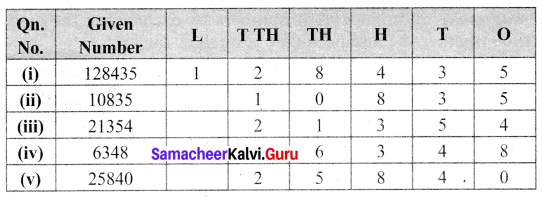The number with more digits is the greater number
Step 1: 128435 is the larger number and 6348 is the least number
Step 2: For the remaining 5 digit numbers we can compare the left-most digits and find 25840 > 21354 > 10835.
The descending order:
128435 > 25840 > 21354 > 10835 > 6348

Question 5.
Write any eight-digit number with 6 in ten lakhs place and 9 in ten-thousandth place.
Solution:
Step (i): Preparing place value chart with 8 digits 6 in ten lakh place and 9 in Ten thousand place
Step (ii): Fill the other places with any of the numbers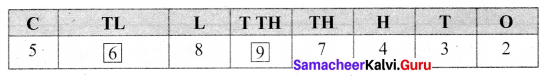The number maybe 56897432. Similarly, we can write many numbers.

Question 6.
Rajan writes a 3-digit number, using the digits 4, 7 and 9. What are the possible numbers he can write?
Solution:
The given digits are 4, 7 and 9.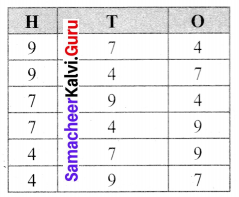Rajan can write 974, 947, 794, 749, 479, 497

Question 7.
The password to access my ATM card includes the digits 9, 4, 6 and 8. It is the smallest 4 digit even number. Find the password of my ATM card.
Solution:
4698Question 8.
Postal Index Number consists of six digits The first three digits are 6, 3 and 1. Make the largest and the smallest Postal Index Number by using the digits 0, 3 and 6 each only once.
Solution:
Given PIN consists of six digits. First, three digits are 6, 3, and 1.
The digits 0, 3 and 6 to be used only once, in the remaining places.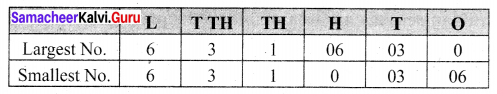Largest Postal Index Number: 631630
Smallest Postal Index Number: 631036

Question 9.
The height (in metres) of the mountains in Tamil Nadu as follows: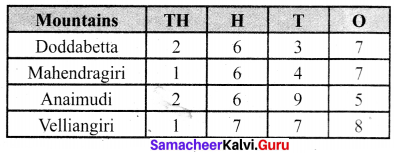(i) Which is the highest mountain listed above?
(ii) Order the mountains from the highest to lowest.
(iii) What is the difference between the heights of the mountains Anaimudi and Mahendragiri?
Solution:
Arranging the numbers in place value chart.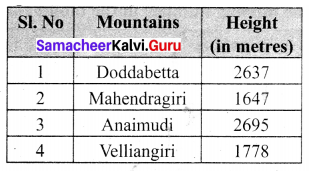(i) The highest mountain is Anaimudi [Comparing left-most digits]
(ii) From the above chart
In thousands place, Doddabetta and Anaimudi have greater value 2.
Comparing digits of 2637 and 2695
2 = 2, 6 = 6, 3 < 9.
2637 < 2695
Again comparing the digits of 1647 and 1778
1 = 1, 6 < 7
1647 < 1778. The required order is 2695 > 2637 > 1778 > 1647.
Anaimudi > Doddabetta > Veliangiri > Mahendragiri
(iii) The height of Anaimudi mountain = 2695 m
The height of Mahendragiri mountain = 1647 m
The Difference = 1048 m

Objective Type Questions

Question 10.
Which list of numbers is in order from the smallest to the largest?
(a) 1468, 1486, 1484
(b) 2345, 2435, 2235
(c) 134205, 134208, 154203
(d) 383553,383548, 383642
Solution:
(c) 134205, 134208, 154203

Question 11.
The Arabian sea has an area of 1491000 square miles. This area lies between which numbers?
(a) 1489000 and 1492540
(b) 1489000 and 1490540
(c) 1490000 and 1490100
(c) 1480000 and 1490000
Solution:
(a) 1489000 and 1492540
Hint: 1489000 < 1491000 < 1492540Question 12.
The chart below shows the number of newspapers sold as per the Indian Readership Survey in 2018. Which could be the missing number in the table?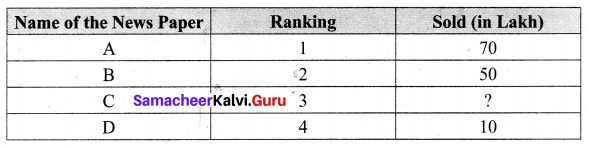(a) 8
(b) 52
(c) 77
(d) 26
Solution:
(d) 26
Hint: 50 > 26 > 10